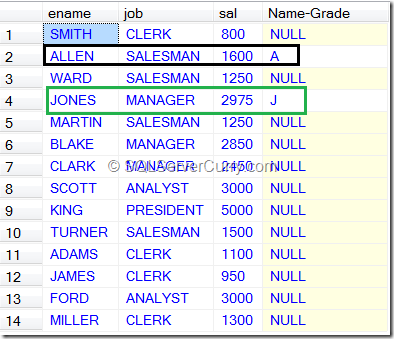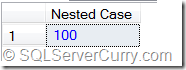### Nested Case Statement in SQL Server

We can nest CASE statements similar to nested ifs that we find in most programming languages.

Let us see an example.

```select ename, job, sal, case  -- Outer Case
when ename like 'A%' then
case when sal >= 1500 then 'A' -- Nested Case
end
when ename like 'J%' then
case when sal >= 2900 then 'J' -- Nested Case
end
From Emp```Image 7-Nested-Case

Limit of nesting a CASE function is up to 10 levels only.

In the following example, the limit of 10 is completely utilized.

```Declare @x int
set @x = 1
Select
case when @x <= 100 then  -- Level 1
case when @x <= 90 then -- Level 2
case when @x <= 80 then -- Level 3
case when @x <= 70 then -- Level 4
case when @x <= 60 then -- Level 5
case when @x <= 50 then -- Level 6
case when @x <= 40 then -- Level 7
case when @x <= 30 then --Level 8
case when @x <= 20 then--Level 9
case when @x<= 10 then--Level 10
100
End End End End End End End End End
End as "Nested Case"
--Ending all levels!```Image 8-Nested-Case-Upto-10-Levels

If nesting is exceeding 10 levels, then SQL Server throws an error.
```Declare @x int
set @x = 1
Select
case when @x <= 100 then  -- Level 1
case when @x <= 90 then -- Level 2
case when @x <= 80 then -- Level 3
case when @x <= 70 then -- Level 4
case when @x <= 60 then -- Level 5
case when @x <= 50 then -- Level 6
case when @x <= 40 then -- Level 7
case when @x <= 30 then --Level 8
case when @x <= 20 then--Level 9
case when @x<= 10 then--Level 10
case when @x <= 11 then -- 11
100
End End End End End End End End End End
End as "Nested Case"```

Msg 125, Level 15, State 4, Line 14
Case expressions may only be nested to level 10.